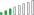cancel
Showing results for
Did you mean:Helper I

## Need help: Cumulative sum function only returns total

Hello,

Edit: Along with the solution I also identified another mistake I made. There was a mismatch between the formatting of my date table and the date column in my fact table (date/time vs date). This cause the relationship between my tables to be crossfiltered instead of being a single one to many -> which in turned made the functions not work.

I have browsed several posts about how to set up a cumulative sum function in the hopes of managing to create one myself, however it does not provide me with the correct output and I am struggling with understanding where I am going wrong.

This is my current formula where OversiktKurs is my Table and [Deltagere Kurs] is the column I would like to calculate the cumulative sum for. I have created a separate date table (dDate), where [Date] has every single date from the start till the end of my fact table. I have also created a relationship between my date table and fact table.

Cumulative sum =
CALCULATE(
SUM(OversiktKurs[Deltagere kurs]),
(dDato[Date]) <= MAX(dDato[Date])
)

I have also tried to include the FILTER function, but that has no impact on the output.
The output gives me the total sum of the column instead of the cumulative. I would assume there is something I'm doing wrong with the date (I have tried to also use the date column in my OversiktKurs column, but this provides me with the same output).Renate

1 ACCEPTED SOLUTIONSuper User

Hi Renate,

Try this as a measure instead, making sure to use dDato fields in visuals, not fact table dates:

``````_cumulativeSum =
VAR __cDate = MAX(dDato[Date])
RETURN
CALCULATE(
SUM(OversiktKurs[Deltagere kurs]),
FILTER(
ALLSELECTED(dDato),
dDato[Date] <= __cDate
)
)``````

Pete

Now accepting Kudos! If my post helped you, why not give it a thumbs-up?

Proud to be a Datanaut!

8 REPLIES 8Super User

Hi Renate,

Try this as a measure instead, making sure to use dDato fields in visuals, not fact table dates:

``````_cumulativeSum =
VAR __cDate = MAX(dDato[Date])
RETURN
CALCULATE(
SUM(OversiktKurs[Deltagere kurs]),
FILTER(
ALLSELECTED(dDato),
dDato[Date] <= __cDate
)
)``````

Pete

Now accepting Kudos! If my post helped you, why not give it a thumbs-up?

Proud to be a Datanaut!Helper I

Hello Pete,

Thank you for the formula!

It seems I may have created a circular dependency as my Date table is created and based on the dates in my fact table;

CALENDAR(DATE(YEAR(MIN(OversiktKurs[Dato])),1,1),
DATE(YEAR(MAX(OversiktKurs[Dato])),12,31)),

I'm not sure if this is ok to do, but when I swap out the formula with the date column in my fact table to test it out, it returns the total sum again.
_cumulativeSum =
VAR __cDate = MAX(OversiktKurs[Dato])
RETURN
CALCULATE(
SUM(OversiktKurs[Deltagere kurs]),
FILTER(
ALLSELECTED(OversiktKurs),
OversiktKurs[Dato] <= __cDate))Super User

Hi Renate,

There's no need to swap out anything in the measure I gave you. It should work exactly as it is.

Please try the measure exactly as I wrote it and let me know how you get on.

Pete

Now accepting Kudos! If my post helped you, why not give it a thumbs-up?

Proud to be a Datanaut!Helper I

I am receiving a circular dependency error - I fear it may be due to how I created my date table.Super User

Are you trying to create this as a calculated column rather than a measure?

You shouln't get a circular dependency when creating a measure as your dDato table would not get recalculated at thatpoint.

Pete

Now accepting Kudos! If my post helped you, why not give it a thumbs-up?

Proud to be a Datanaut!Helper I

You are correct, it completely slipped my mind.

It worked now, thank you so much for your help.

Best regards,

RenateResolver III

Hi @RenateBK ,

I always use earlier to calculate a cumulative sum in combination with a date value. Earlier is more flexible than the max function you are currently using. Please check this for an example on calculating a cumulative sum using the earlier function.Helper I

Thank you for the response, I tried to use the EARLIER function, but I am receiving an error saying that "the syntax for date is wrong" and the error message ending with 6 parenteses instead of 3.If I try to use it without .Date

Cumulative sum =
CALCULATE(
SUM(OversiktKurs[Deltagere kurs]),
FILTER(OversiktKurs,dDato[Date] <= EARLIER(dDato[Date]))
)

I get the error "A single value for column 'Tangoe Owner Email' in table 'Monthly Billing' cannot be determined. This can happen when a measure formula refers to a column that contains many values without specifying an aggregation such as min, max, count, or sum to get a single result."